Author: Pauli Virtanen

Numpy is at the base of Python’s scientific stack of tools. Its purpose to implement efficient operations on many items in a block of memory. Understanding how it works in detail helps in making efficient use of its flexibility, taking useful shortcuts.

This section covers:

• Anatomy of Numpy arrays, and its consequences. Tips and tricks.
• Universal functions: what, why, and what to do if you want a new one.
• Integration with other tools: Numpy offers several ways to wrap any data in an ndarray, without unnecessary copies.
• Recently added features, and what’s in them: PEP 3118 buffers, generalized ufuncs, ...

Prerequisites

• Numpy
• Cython
• Pillow (Python imaging library, used in a couple of examples)

Tip

In this section, numpy will be imported as follows:

>>> import numpy as np


## 2.2.1. Life of ndarray¶

### 2.2.1.1. It’s...¶

ndarray =

block of memory + indexing scheme + data type descriptor

• raw data
• how to locate an element
• how to interpret an element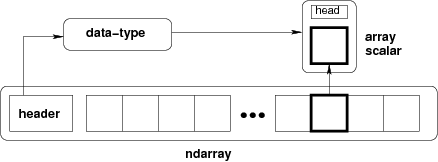typedef struct PyArrayObject {

/* Block of memory */
char *data;

/* Data type descriptor */
PyArray_Descr *descr;

/* Indexing scheme */
int nd;
npy_intp *dimensions;
npy_intp *strides;

/* Other stuff */
PyObject *base;
int flags;
PyObject *weakreflist;
} PyArrayObject;


### 2.2.1.2. Block of memory¶

>>> x = np.array([1, 2, 3], dtype=np.int32)
>>> x.data
<... at ...>
>>> str(x.data)
'\x01\x00\x00\x00\x02\x00\x00\x00\x03\x00\x00\x00'


>>> x.__array_interface__['data']
64803824


The whole __array_interface__:

>>> x.__array_interface__
{'data': (35828928, False),
'descr': [('', '<i4')],
'shape': (4,),
'strides': None,
'typestr': '<i4',
'version': 3}


Reminder: two ndarrays may share the same memory:

>>> x = np.array([1, 2, 3, 4])
>>> y = x[:-1]
>>> x = 9
>>> y
array([9, 2, 3])


Memory does not need to be owned by an ndarray:

>>> x = b'1234'      # The 'b' is for "bytes", necessary in Python 3


x is a string (in Python 3 a bytes), we can represent its data as an array of ints:

>>> y = np.frombuffer(x, dtype=np.int8)
>>> y.data
<... at ...>
>>> y.base is x
True

>>> y.flags
C_CONTIGUOUS : True
F_CONTIGUOUS : True
OWNDATA : False
WRITEABLE : False
ALIGNED : True
UPDATEIFCOPY : False


The owndata and writeable flags indicate status of the memory block.

### 2.2.1.3. Data types¶

#### 2.2.1.3.1. The descriptor¶

dtype describes a single item in the array:

 type scalar type of the data, one of: int8, int16, float64, et al. (fixed size) str, unicode, void (flexible size) itemsize size of the data block byteorder byte order: big-endian > / little-endian < / not applicable | fields sub-dtypes, if it’s a structured data type shape shape of the array, if it’s a sub-array
>>> np.dtype(int).type
<type 'numpy.int64'>
>>> np.dtype(int).itemsize
8
>>> np.dtype(int).byteorder
'='


#### 2.2.1.3.2. Example: reading .wav files¶

 chunk_id "RIFF" chunk_size 4-byte unsigned little-endian integer format "WAVE" fmt_id "fmt " fmt_size 4-byte unsigned little-endian integer audio_fmt 2-byte unsigned little-endian integer num_channels 2-byte unsigned little-endian integer sample_rate 4-byte unsigned little-endian integer byte_rate 4-byte unsigned little-endian integer block_align 2-byte unsigned little-endian integer bits_per_sample 2-byte unsigned little-endian integer data_id "data" data_size 4-byte unsigned little-endian integer
• 44-byte block of raw data (in the beginning of the file)
• ... followed by data_size bytes of actual sound data.

The .wav file header as a Numpy structured data type:

>>> wav_header_dtype = np.dtype([
...     ("chunk_id", (bytes, 4)), # flexible-sized scalar type, item size 4
...     ("chunk_size", "<u4"),    # little-endian unsigned 32-bit integer
...     ("format", "S4"),         # 4-byte string
...     ("fmt_id", "S4"),
...     ("fmt_size", "<u4"),
...     ("audio_fmt", "<u2"),     #
...     ("num_channels", "<u2"),  # .. more of the same ...
...     ("sample_rate", "<u4"),   #
...     ("byte_rate", "<u4"),
...     ("block_align", "<u2"),
...     ("bits_per_sample", "<u2"),
...     ("data_id", ("S1", (2, 2))), # sub-array, just for fun!
...     ("data_size", "u4"),
...     #
...     # the sound data itself cannot be represented here:
...     # it does not have a fixed size
...    ])


>>> wav_header_dtype['format']
dtype('S4')
dict_proxy({'block_align': (dtype('uint16'), 32), 'format': (dtype('S4'), 8), 'data_id': (dtype(('S1', (2, 2))), 36), 'fmt_id': (dtype('S4'), 12), 'byte_rate': (dtype('uint32'), 28), 'chunk_id': (dtype('S4'), 0), 'num_channels': (dtype('uint16'), 22), 'sample_rate': (dtype('uint32'), 24), 'bits_per_sample': (dtype('uint16'), 34), 'chunk_size': (dtype('uint32'), 4), 'fmt_size': (dtype('uint32'), 16), 'data_size': (dtype('uint32'), 40), 'audio_fmt': (dtype('uint16'), 20)})
(dtype('S4'), 8)

• The first element is the sub-dtype in the structured data, corresponding to the name format
• The second one is its offset (in bytes) from the beginning of the item

Exercise

Mini-exercise, make a “sparse” dtype by using offsets, and only some of the fields:

>>> wav_header_dtype = np.dtype(dict(
...   names=['format', 'sample_rate', 'data_id'],
...   offsets=[offset_1, offset_2, offset_3], # counted from start of structure in bytes
...   formats=list of dtypes for each of the fields,
... ))


and use that to read the sample rate, and data_id (as sub-array).

>>> f = open('data/test.wav', 'r')
>>> f.close()
[ ('RIFF', 17402L, 'WAVE', 'fmt ', 16L, 1, 1, 16000L, 32000L, 2, 16, [['d', 'a'], ['t', 'a']], 17366L)]
array(, dtype=uint32)


Let’s try accessing the sub-array:

>>> wav_header['data_id']
array([[['d', 'a'],
['t', 'a']]],
dtype='|S1')
(1,)
(1, 2, 2)


When accessing sub-arrays, the dimensions get added to the end!

Note

There are existing modules such as wavfile, audiolab, etc. for loading sound data...

#### 2.2.1.3.3. Casting and re-interpretation/views¶

casting

• on assignment
• on array construction
• on arithmetic
• etc.
• and manually: .astype(dtype)

data re-interpretation

• manually: .view(dtype)
##### 2.2.1.3.3.1. Casting¶
• Casting in arithmetic, in nutshell:

• only type (not value!) of operands matters
• largest “safe” type able to represent both is picked
• scalars can “lose” to arrays in some situations
• Casting in general copies data:

>>> x = np.array([1, 2, 3, 4], dtype=np.float)
>>> x
array([ 1.,  2.,  3.,  4.])
>>> y = x.astype(np.int8)
>>> y
array([1, 2, 3, 4], dtype=int8)
>>> y + 1
array([2, 3, 4, 5], dtype=int8)
>>> y + 256
array([257, 258, 259, 260], dtype=int16)
>>> y + 256.0
array([ 257.,  258.,  259.,  260.])
>>> y + np.array(, dtype=np.int32)
array([257, 258, 259, 260], dtype=int32)

• Casting on setitem: dtype of the array is not changed on item assignment:

>>> y[:] = y + 1.5
>>> y
array([2, 3, 4, 5], dtype=int8)


Note

Exact rules: see numpy documentation

##### 2.2.1.3.3.2. Re-interpretation / viewing¶
• Data block in memory (4 bytes)

 0x01 || 0x02 || 0x03 || 0x04
• 4 of uint8, OR,
• 4 of int8, OR,
• 2 of int16, OR,
• 1 of int32, OR,
• 1 of float32, OR,
• ...

How to switch from one to another?

1. Switch the dtype:

>>> x = np.array([1, 2, 3, 4], dtype=np.uint8)
>>> x.dtype = "<i2"
>>> x
array([ 513, 1027], dtype=int16)
>>> 0x0201, 0x0403
(513, 1027)

 0x01 0x02 || 0x03 0x04

Note

little-endian: least significant byte is on the left in memory

1. Create a new view:

>>> y = x.view("<i4")
>>> y
array(, dtype=int32)
>>> 0x04030201
67305985

 0x01 0x02 0x03 0x04

Note

• .view() makes views, does not copy (or alter) the memory block

• only changes the dtype (and adjusts array shape):

>>> x = 5
>>> y
array(, dtype=int32)
>>> y.base is x
True


Mini-exercise: data re-interpretation

view-colors.py

You have RGBA data in an array:

>>> x = np.zeros((10, 10, 4), dtype=np.int8)
>>> x[:, :, 0] = 1
>>> x[:, :, 1] = 2
>>> x[:, :, 2] = 3
>>> x[:, :, 3] = 4


where the last three dimensions are the R, B, and G, and alpha channels.

How to make a (10, 10) structured array with field names ‘r’, ‘g’, ‘b’, ‘a’ without copying data?

>>> y = ...

>>> assert (y['r'] == 1).all()
>>> assert (y['g'] == 2).all()
>>> assert (y['b'] == 3).all()
>>> assert (y['a'] == 4).all()


Solution

...

Warning

Another array taking exactly 4 bytes of memory:

>>> y = np.array([[1, 3], [2, 4]], dtype=np.uint8).transpose()
>>> x = y.copy()
>>> x
array([[1, 2],
[3, 4]], dtype=uint8)
>>> y
array([[1, 2],
[3, 4]], dtype=uint8)
>>> x.view(np.int16)
array([[ 513],
], dtype=int16)
>>> 0x0201, 0x0403
(513, 1027)
>>> y.view(np.int16)
array([[ 769, 1026]], dtype=int16)

• What happened?
• ... we need to look into what x[0,1] actually means
>>> 0x0301, 0x0402
(769, 1026)


### 2.2.1.4. Indexing scheme: strides¶

#### 2.2.1.4.1. Main point¶

The question:

>>> x = np.array([[1, 2, 3],
...               [4, 5, 6],
...               [7, 8, 9]], dtype=np.int8)
>>> str(x.data)
'\x01\x02\x03\x04\x05\x06\x07\x08\t'

At which byte in x.data does the item x[1, 2] begin?


• strides: the number of bytes to jump to find the next element
• 1 stride per dimension
  >>> x.strides
(3, 1)
>>> byte_offset = 3*1 + 1*2   # to find x[1, 2]
>>> x.flat[byte_offset]
6
>>> x[1, 2]
6

- simple, **flexible**

##### 2.2.1.4.1.1. C and Fortran order¶
>>> x = np.array([[1, 2, 3],
...               [4, 5, 6]], dtype=np.int16, order='C')
>>> x.strides
(6, 2)
>>> str(x.data)
'\x01\x00\x02\x00\x03\x00\x04\x00\x05\x00\x06\x00'

• Need to jump 6 bytes to find the next row
• Need to jump 2 bytes to find the next column
>>> y = np.array(x, order='F')
>>> y.strides
(2, 4)
>>> str(y.data)
'\x01\x00\x04\x00\x02\x00\x05\x00\x03\x00\x06\x00'

• Need to jump 2 bytes to find the next row
• Need to jump 4 bytes to find the next column
• Similarly to higher dimensions:

• C: last dimensions vary fastest (= smaller strides)
• F: first dimensions vary fastest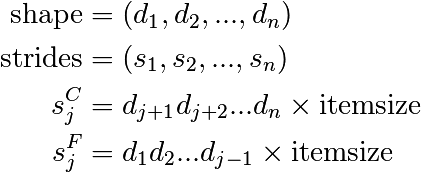Note

Now we can understand the behavior of .view():

>>> y = np.array([[1, 3], [2, 4]], dtype=np.uint8).transpose()
>>> x = y.copy()


Transposition does not affect the memory layout of the data, only strides

>>> x.strides
(2, 1)
>>> y.strides
(1, 2)

>>> str(x.data)
'\x01\x02\x03\x04'
>>> str(y.data)
'\x01\x03\x02\x04'

• the results are different when interpreted as 2 of int16
• .copy() creates new arrays in the C order (by default)
##### 2.2.1.4.1.2. Slicing with integers¶
• Everything can be represented by changing only shape, strides, and possibly adjusting the data pointer!
• Never makes copies of the data
>>> x = np.array([1, 2, 3, 4, 5, 6], dtype=np.int32)
>>> y = x[::-1]
>>> y
array([6, 5, 4, 3, 2, 1], dtype=int32)
>>> y.strides
(-4,)

>>> y = x[2:]
>>> y.__array_interface__['data'] - x.__array_interface__['data']
8

>>> x = np.zeros((10, 10, 10), dtype=np.float)
>>> x.strides
(800, 80, 8)
>>> x[::2,::3,::4].strides
(1600, 240, 32)

• Similarly, transposes never make copies (it just swaps strides):

>>> x = np.zeros((10, 10, 10), dtype=np.float)
>>> x.strides
(800, 80, 8)
>>> x.T.strides
(8, 80, 800)


But: not all reshaping operations can be represented by playing with strides:

>>> a = np.arange(6, dtype=np.int8).reshape(3, 2)
>>> b = a.T
>>> b.strides
(1, 2)


So far, so good. However:

>>> str(a.data)
'\x00\x01\x02\x03\x04\x05'
>>> b
array([[0, 2, 4],
[1, 3, 5]], dtype=int8)
>>> c = b.reshape(3*2)
>>> c
array([0, 2, 4, 1, 3, 5], dtype=int8)


Here, there is no way to represent the array c given one stride and the block of memory for a. Therefore, the reshape operation needs to make a copy here.

#### 2.2.1.4.2. Example: fake dimensions with strides¶

Stride manipulation

>>> from numpy.lib.stride_tricks import as_strided
>>> help(as_strided)
as_strided(x, shape=None, strides=None)
Make an ndarray from the given array with the given shape and strides


Warning

as_strided does not check that you stay inside the memory block bounds...

>>> x = np.array([1, 2, 3, 4], dtype=np.int16)
>>> as_strided(x, strides=(2*2, ), shape=(2, ))
array([1, 3], dtype=int16)
>>> x[::2]
array([1, 3], dtype=int16)


stride-fakedims.py

Exercise

array([1, 2, 3, 4], dtype=np.int8)

-> array([[1, 2, 3, 4],
[1, 2, 3, 4],
[1, 2, 3, 4]], dtype=np.int8)


using only as_strided.:

Hint: byte_offset = stride*index + stride*index + ...


Spoiler

...

• Doing something useful with it: outer product of [1, 2, 3, 4] and [5, 6, 7]
>>> x = np.array([1, 2, 3, 4], dtype=np.int16)
>>> x2 = as_strided(x, strides=(0, 1*2), shape=(3, 4))
>>> x2
array([[1, 2, 3, 4],
[1, 2, 3, 4],
[1, 2, 3, 4]], dtype=int16)

>>> y = np.array([5, 6, 7], dtype=np.int16)
>>> y2 = as_strided(y, strides=(1*2, 0), shape=(3, 4))
>>> y2
array([[5, 5, 5, 5],
[6, 6, 6, 6],
[7, 7, 7, 7]], dtype=int16)

>>> x2 * y2
array([[ 5, 10, 15, 20],
[ 6, 12, 18, 24],
[ 7, 14, 21, 28]], dtype=int16)


... seems somehow familiar ...

>>> x = np.array([1, 2, 3, 4], dtype=np.int16)
>>> y = np.array([5, 6, 7], dtype=np.int16)
>>> x[np.newaxis,:] * y[:,np.newaxis]
array([[ 5, 10, 15, 20],
[ 6, 12, 18, 24],
[ 7, 14, 21, 28]], dtype=int16)

• Internally, array broadcasting is indeed implemented using 0-strides.

#### 2.2.1.4.4. More tricks: diagonals¶

stride-diagonals.py

Challenge

• Pick diagonal entries of the matrix: (assume C memory order):

>>> x = np.array([[1, 2, 3],
...               [4, 5, 6],
...               [7, 8, 9]], dtype=np.int32)

>>> x_diag = as_strided(x, shape=(3,), strides=(???,))

• Pick the first super-diagonal entries [2, 6].

• And the sub-diagonals?

(Hint to the last two: slicing first moves the point where striding
starts from.)

Solution

...

stride-diagonals.py

Challenge

Compute the tensor trace:

>>> x = np.arange(5*5*5*5).reshape(5, 5, 5, 5)
>>> s = 0
>>> for i in range(5):
...    for j in range(5):
...       s += x[j, i, j, i]


by striding, and using sum() on the result.

>>> y = as_strided(x, shape=(5, 5), strides=(TODO, TODO))
>>> s2 = ...
>>> assert s == s2


Solution

...

#### 2.2.1.4.5. CPU cache effects¶

Memory layout can affect performance:

In : x = np.zeros((20000,))

In : y = np.zeros((20000*67,))[::67]

In : x.shape, y.shape
((20000,), (20000,))

In : %timeit x.sum()
100000 loops, best of 3: 0.180 ms per loop

In : %timeit y.sum()
100000 loops, best of 3: 2.34 ms per loop

In : x.strides, y.strides
((8,), (536,))


Smaller strides are faster?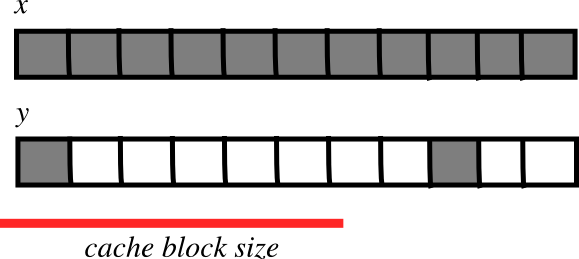• CPU pulls data from main memory to its cache in blocks
• If many array items consecutively operated on fit in a single block (small stride):
•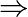fewer transfers needed
•faster

numexpr is designed to mitigate cache effects in array computing.

#### 2.2.1.4.6. Example: inplace operations (caveat emptor)¶

• Sometimes,

>>> a -= b


is not the same as

>>> a -= b.copy()

>>> x = np.array([[1, 2], [3, 4]])
>>> x -= x.transpose()
>>> x
array([[ 0, -1],
[ 4,  0]])

>>> y = np.array([[1, 2], [3, 4]])
>>> y -= y.T.copy()
>>> y
array([[ 0, -1],
[ 1,  0]])

• x and x.transpose() share data
• x -= x.transpose() modifies the data element-by-element...
• because x and x.transpose() have different striding, modified data re-appears on the RHS

### 2.2.1.5. Findings in dissection¶• memory block: may be shared, .base, .data
• data type descriptor: structured data, sub-arrays, byte order, casting, viewing, .astype(), .view()
• strided indexing: strides, C/F-order, slicing w/ integers, as_strided, broadcasting, stride tricks, diag, CPU cache coherence

## 2.2.2. Universal functions¶

### 2.2.2.1. What they are?¶

• Ufunc performs and elementwise operation on all elements of an array.

Examples:

np.add, np.subtract, scipy.special.*, ...

• Automatically support: broadcasting, casting, ...

• The author of an ufunc only has to supply the elementwise operation, Numpy takes care of the rest.

• The elementwise operation needs to be implemented in C (or, e.g., Cython)

#### 2.2.2.1.1. Parts of an Ufunc¶

1. Provided by user

void ufunc_loop(void **args, int *dimensions, int *steps, void *data)
{
/*
* int8 output = elementwise_function(int8 input_1, int8 input_2)
*
* This function must compute the ufunc for many values at once,
* in the way shown below.
*/
char *input_1 = (char*)args;
char *input_2 = (char*)args;
char *output = (char*)args;
int i;

for (i = 0; i < dimensions; ++i) {
*output = elementwise_function(*input_1, *input_2);
input_1 += steps;
input_2 += steps;
output += steps;
}
}

2. The Numpy part, built by

char types

types = NPY_BYTE   /* type of first input arg */
types = NPY_BYTE   /* type of second input arg */
types = NPY_BYTE   /* type of third input arg */

PyObject *python_ufunc = PyUFunc_FromFuncAndData(
ufunc_loop,
NULL,
types,
1, /* ntypes */
2, /* num_inputs */
1, /* num_outputs */
identity_element,
name,
docstring,
unused)

• A ufunc can also support multiple different input-output type combinations.

#### 2.2.2.1.2. Making it easier¶

1. ufunc_loop is of very generic form, and Numpy provides pre-made ones

 PyUfunc_f_f float elementwise_func(float input_1) PyUfunc_ff_f float elementwise_func(float input_1, float input_2) PyUfunc_d_d double elementwise_func(double input_1) PyUfunc_dd_d double elementwise_func(double input_1, double input_2) PyUfunc_D_D elementwise_func(npy_cdouble *input, npy_cdouble* output) PyUfunc_DD_D elementwise_func(npy_cdouble *in1, npy_cdouble *in2, npy_cdouble* out)
• Only elementwise_func needs to be supplied
• ... except when your elementwise function is not in one of the above forms

### 2.2.2.2. Exercise: building an ufunc from scratch¶

The Mandelbrot fractal is defined by the iteration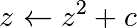where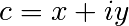is a complex number. This iteration is repeated – if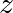stays finite no matter how long the iteration runs,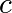belongs to the Mandelbrot set.

• Make ufunc called mandel(z0, c) that computes:

z = z0
for k in range(iterations):
z = z*z + c


say, 100 iterations or until z.real**2 + z.imag**2 > 1000. Use it to determine which c are in the Mandelbrot set.

• Our function is a simple one, so make use of the PyUFunc_* helpers.

• Write it in Cython

mandel.pyx, mandelplot.py

 PyUfunc_f_f float elementwise_func(float input_1) PyUfunc_ff_f float elementwise_func(float input_1, float input_2) PyUfunc_d_d double elementwise_func(double input_1) PyUfunc_dd_d double elementwise_func(double input_1, double input_2) PyUfunc_D_D elementwise_func(complex_double *input, complex_double* output) PyUfunc_DD_D elementwise_func(complex_double *in1, complex_double *in2, complex_double* out)

Type codes:

NPY_BOOL, NPY_BYTE, NPY_UBYTE, NPY_SHORT, NPY_USHORT, NPY_INT, NPY_UINT,
NPY_LONG, NPY_ULONG, NPY_LONGLONG, NPY_ULONGLONG, NPY_FLOAT, NPY_DOUBLE,
NPY_LONGDOUBLE, NPY_CFLOAT, NPY_CDOUBLE, NPY_CLONGDOUBLE, NPY_DATETIME,
NPY_TIMEDELTA, NPY_OBJECT, NPY_STRING, NPY_UNICODE, NPY_VOID


### 2.2.2.3. Solution: building an ufunc from scratch¶

# The elementwise function
# ------------------------

cdef void mandel_single_point(double complex *z_in,
double complex *c_in,
double complex *z_out) nogil:
#
# The Mandelbrot iteration
#

#
# Some points of note:
#
# - It's *NOT* allowed to call any Python functions here.
#
#   The Ufunc loop runs with the Python Global Interpreter Lock released.
#   Hence, the nogil.
#
# - And so all local variables must be declared with cdef
#
# - Note also that this function receives *pointers* to the data;
#   the "traditional" solution to passing complex variables around
#

cdef double complex z = z_in
cdef double complex c = c_in
cdef int k  # the integer we use in the for loop

# Straightforward iteration

for k in range(100):
z = z*z + c
if z.real**2 + z.imag**2 > 1000:
break

# Return the answer for this point
z_out = z

# Boilerplate Cython definitions
#
# You don't really need to read this part, it just pulls in
# stuff from the Numpy C headers.
# ----------------------------------------------------------

cdef extern from "numpy/arrayobject.h":
void import_array()
ctypedef int npy_intp
cdef enum NPY_TYPES:
NPY_CDOUBLE

cdef extern from "numpy/ufuncobject.h":
void import_ufunc()
ctypedef void (*PyUFuncGenericFunction)(char**, npy_intp*, npy_intp*, void*)
object PyUFunc_FromFuncAndData(PyUFuncGenericFunction* func, void** data,
char* types, int ntypes, int nin, int nout,
int identity, char* name, char* doc, int c)

void PyUFunc_DD_D(char**, npy_intp*, npy_intp*, void*)

# Required module initialization
# ------------------------------

import_array()
import_ufunc()

# The actual ufunc declaration
# ----------------------------

cdef PyUFuncGenericFunction loop_func
cdef char input_output_types
cdef void *elementwise_funcs

loop_func = PyUFunc_DD_D

input_output_types = NPY_CDOUBLE
input_output_types = NPY_CDOUBLE
input_output_types = NPY_CDOUBLE

elementwise_funcs = <void*>mandel_single_point

mandel = PyUFunc_FromFuncAndData(
loop_func,
elementwise_funcs,
input_output_types,
1, # number of supported input types
2, # number of input args
1, # number of output args
0, # identity element, never mind this
"mandel", # function name
"mandel(z, c) -> computes iterated z*z + c", # docstring
0 # unused
)

"""
Plot Mandelbrot
================

Plot the Mandelbrot ensemble.

"""

import numpy as np
import mandel
x = np.linspace(-1.7, 0.6, 1000)
y = np.linspace(-1.4, 1.4, 1000)
c = x[None,:] + 1j*y[:,None]
z = mandel.mandel(c, c)

import matplotlib.pyplot as plt
plt.imshow(abs(z)**2 < 1000, extent=[-1.7, 0.6, -1.4, 1.4])
plt.gray()
plt.show()Note

Most of the boilerplate could be automated by these Cython modules:

http://wiki.cython.org/MarkLodato/CreatingUfuncs

Several accepted input types

E.g. supporting both single- and double-precision versions

cdef void mandel_single_point(double complex *z_in,
double complex *c_in,
double complex *z_out) nogil:
...

cdef void mandel_single_point_singleprec(float complex *z_in,
float complex *c_in,
float complex *z_out) nogil:
...

cdef PyUFuncGenericFunction loop_funcs
cdef char input_output_types[3*2]
cdef void *elementwise_funcs[1*2]

loop_funcs = PyUFunc_DD_D
input_output_types = NPY_CDOUBLE
input_output_types = NPY_CDOUBLE
input_output_types = NPY_CDOUBLE
elementwise_funcs = <void*>mandel_single_point

loop_funcs = PyUFunc_FF_F
input_output_types = NPY_CFLOAT
input_output_types = NPY_CFLOAT
input_output_types = NPY_CFLOAT
elementwise_funcs = <void*>mandel_single_point_singleprec

mandel = PyUFunc_FromFuncAndData(
loop_func,
elementwise_funcs,
input_output_types,
2, # number of supported input types   <----------------
2, # number of input args
1, # number of output args
0, # identity element, never mind this
"mandel", # function name
"mandel(z, c) -> computes iterated z*z + c", # docstring
0 # unused
)


### 2.2.2.4. Generalized ufuncs¶

ufunc

output = elementwise_function(input)

Both output and input can be a single array element only.

generalized ufunc

output and input can be arrays with a fixed number of dimensions

For example, matrix trace (sum of diag elements):

input shape = (n, n)
output shape = ()      i.e.  scalar

(n, n) -> ()


Matrix product:

input_1 shape = (m, n)
input_2 shape = (n, p)
output shape  = (m, p)

(m, n), (n, p) -> (m, p)

• This is called the “signature” of the generalized ufunc
• The dimensions on which the g-ufunc acts, are “core dimensions”

Status in Numpy

• g-ufuncs are in Numpy already ...
• new ones can be created with PyUFunc_FromFuncAndDataAndSignature
• ... but we don’t ship with public g-ufuncs, except for testing, ATM
>>> import numpy.core.umath_tests as ut
>>> ut.matrix_multiply.signature
'(m,n),(n,p)->(m,p)'

>>> x = np.ones((10, 2, 4))
>>> y = np.ones((10, 4, 5))
>>> ut.matrix_multiply(x, y).shape
(10, 2, 5)

• the last two dimensions became core dimensions, and are modified as per the signature
• otherwise, the g-ufunc operates “elementwise”
• matrix multiplication this way could be useful for operating on many small matrices at once

Generalized ufunc loop

Matrix multiplication (m,n),(n,p) -> (m,p)

void gufunc_loop(void **args, int *dimensions, int *steps, void *data)
{
char *input_1 = (char*)args;  /* these are as previously */
char *input_2 = (char*)args;
char *output = (char*)args;

int input_1_stride_m = steps;  /* strides for the core dimensions */
int input_1_stride_n = steps;  /* are added after the non-core */
int input_2_strides_n = steps; /* steps */
int input_2_strides_p = steps;
int output_strides_n = steps;
int output_strides_p = steps;

int m = dimension; /* core dimensions are added after */
int n = dimension; /* the main dimension; order as in */
int p = dimension; /* signature */

int i;

for (i = 0; i < dimensions; ++i) {
matmul_for_strided_matrices(input_1, input_2, output,
strides for each array...);

input_1 += steps;
input_2 += steps;
output += steps;
}
}


## 2.2.3. Interoperability features¶

### 2.2.3.1. Sharing multidimensional, typed data¶

Suppose you

1. Write a library than handles (multidimensional) binary data,
2. Want to make it easy to manipulate the data with Numpy, or whatever other library,
3. ... but would not like to have Numpy as a dependency.

Currently, 3 solutions:

1. the “old” buffer interface
2. the array interface
3. the “new” buffer interface (PEP 3118)

### 2.2.3.2. The old buffer protocol¶

• Only 1-D buffers
• No data type information
• C-level interface; PyBufferProcs tp_as_buffer in the type object
• But it’s integrated into Python (e.g. strings support it)

Mini-exercise using Pillow (Python Imaging Library):

pilbuffer.py

>>> from PIL import Image
>>> data = np.zeros((200, 200, 4), dtype=np.int8)
>>> data[:, :] = [255, 0, 0, 255] # Red
>>> # In PIL, RGBA images consist of 32-bit integers whose bytes are [RR,GG,BB,AA]
>>> data = data.view(np.int32).squeeze()
>>> img = Image.frombuffer("RGBA", (200, 200), data, "raw", "RGBA", 0, 1)
>>> img.save('test.png')


Q:

Check what happens if data is now modified, and img saved again.

### 2.2.3.3. The old buffer protocol¶

"""
From buffer
============

Show how to exchange data between numpy and a library that only knows
the buffer interface.
"""

import numpy as np
import Image

# Let's make a sample image, RGBA format

x = np.zeros((200, 200, 4), dtype=np.int8)

x[:,:,0] = 254 # red
x[:,:,3] = 255 # opaque

data = x.view(np.int32) # Check that you understand why this is OK!

img = Image.frombuffer("RGBA", (200, 200), data)
img.save('test.png')

#
# Modify the original data, and save again.
#
# It turns out that PIL, which knows next to nothing about Numpy,
# happily shares the same data.
#

x[:,:,1] = 254
img.save('test2.png')### 2.2.3.4. Array interface protocol¶

• Multidimensional buffers
• Data type information present
• Numpy-specific approach; slowly deprecated (but not going away)
• Not integrated in Python otherwise

>>> x = np.array([[1, 2], [3, 4]])
>>> x.__array_interface__
'descr': [('', '<i4')],          # data type descriptor
'typestr': '<i4',                # same, in another form
'strides': None,                 # strides; or None if in C-order
'shape': (2, 2),
'version': 3,
}

::
>>> from PIL import Image
>>> img = Image.open('data/test.png')
>>> img.__array_interface__
{'data': ...,
'shape': (200, 200, 4),
'typestr': '|u1'}
>>> x = np.asarray(img)
>>> x.shape
(200, 200, 4)


Note

A more C-friendly variant of the array interface is also defined.

## 2.2.4. Array siblings: chararray, maskedarray, matrix¶

### 2.2.4.1. chararray: vectorized string operations¶

>>> x = np.array(['a', '  bbb', '  ccc']).view(np.chararray)
>>> x.lstrip(' ')
chararray(['a', 'bbb', 'ccc'],
dtype='...')
>>> x.upper()
chararray(['A', '  BBB', '  CCC'],
dtype='...')


Note

.view() has a second meaning: it can make an ndarray an instance of a specialized ndarray subclass

Masked arrays are arrays that may have missing or invalid entries.

For example, suppose we have an array where the fourth entry is invalid:

>>> x = np.array([1, 2, 3, -99, 5])


One way to describe this is to create a masked array:

>>> mx = np.ma.masked_array(x, mask=[0, 0, 0, 1, 0])
>>> mx
masked_array(data = [1 2 3 -- 5],
mask = [False False False  True False],
fill_value = 999999)


>>> mx.mean()
2.75
>>> np.mean(mx)
2.75


Warning

Not all Numpy functions respect masks, for instance np.dot, so check the return types.

The masked_array returns a view to the original array:

>>> mx = 9
>>> x
array([  1,   9,   3, -99,   5])


You can modify the mask by assigning:

>>> mx = np.ma.masked
>>> mx
masked_array(data = [1 -- 3 -- 5],
mask = [False  True False  True False],
fill_value = 999999)


The mask is cleared on assignment:

>>> mx = 9
>>> mx
masked_array(data = [1 9 3 -- 5],
mask = [False False False  True False],
fill_value = 999999)


The mask is also available directly:

>>> mx.mask
array([False, False, False,  True, False], dtype=bool)


The masked entries can be filled with a given value to get an usual array back:

>>> x2 = mx.filled(-1)
>>> x2
array([ 1,  9,  3, -1,  5])


The mask can also be cleared:

>>> mx.mask = np.ma.nomask
>>> mx
masked_array(data = [1 9 3 -99 5],
mask = [False False False False False],
fill_value = 999999)


#### 2.2.4.2.2. Domain-aware functions¶

The masked array package also contains domain-aware functions:

>>> np.ma.log(np.array([1, 2, -1, -2, 3, -5]))
masked_array(data = [0.0 0.6931471805599453 -- -- 1.0986122886681098 --],
mask = [False False  True  True False  True],
fill_value = 1e+20)


Note

Streamlined and more seamless support for dealing with missing data in arrays is making its way into Numpy 1.7. Stay tuned!

Canadian rangers were distracted when counting hares and lynxes in 1903-1910 and 1917-1918, and got the numbers are wrong. (Carrot farmers stayed alert, though.) Compute the mean populations over time, ignoring the invalid numbers.

>>> data = np.loadtxt('data/populations.txt')
>>> year = data[:, 0]

>>> bad_years = (((year >= 1903) & (year <= 1910))
...            | ((year >= 1917) & (year <= 1918)))
>>> # '&' means 'and' and '|' means 'or'

>>> populations.mean(axis=0)
fill_value = 1e+20)

>>> populations.std(axis=0)
fill_value = 1e+20)


>>> plt.plot(year, populations, 'o-')
[<matplotlib.lines.Line2D object at ...>, ...]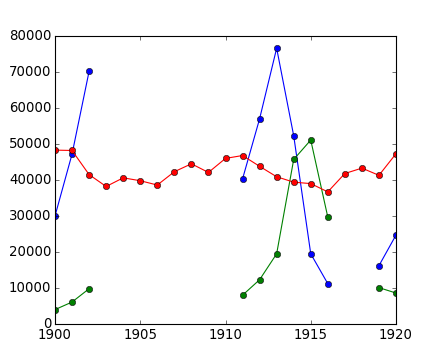### 2.2.4.3. recarray: purely convenience¶

>>> arr = np.array([('a', 1), ('b', 2)], dtype=[('x', 'S1'), ('y', int)])
>>> arr2 = arr.view(np.recarray)
>>> arr2.x
chararray(['a', 'b'],
dtype='|S1')
>>> arr2.y
array([1, 2])


### 2.2.4.4. matrix: convenience?¶

• always 2-D
• * is the matrix product, not the elementwise one
>>> np.matrix([[1, 0], [0, 1]]) * np.matrix([[1, 2], [3, 4]])
matrix([[1, 2],
[3, 4]])


## 2.2.5. Summary¶

• Anatomy of the ndarray: data, dtype, strides.
• Universal functions: elementwise operations, how to make new ones
• Ndarray subclasses
• Various buffer interfaces for integration with other tools
• Recent additions: PEP 3118, generalized ufuncs

## 2.2.6. Contributing to Numpy/Scipy¶

### 2.2.6.1. Why¶

• “There’s a bug?”
• “I don’t understand what this is supposed to do?”
• “I have this fancy code. Would you like to have it?”
• “I’d like to help! What can I do?”

### 2.2.6.2. Reporting bugs¶

#### 2.2.6.2.1. Good bug report¶

Title: numpy.random.permutations fails for non-integer arguments

I'm trying to generate random permutations, using numpy.random.permutations

When calling numpy.random.permutation with non-integer arguments
it fails with a cryptic error message::

>>> np.random.permutation(12)
array([ 6, 11,  4, 10,  2,  8,  1,  7,  9,  3,  0,  5])
>>> np.random.permutation(12.)
Traceback (most recent call last):
File "<stdin>", line 1, in <module>
File "mtrand.pyx", line 3311, in mtrand.RandomState.permutation
File "mtrand.pyx", line 3254, in mtrand.RandomState.shuffle
TypeError: len() of unsized object

This also happens with long arguments, and so
np.random.permutation(X.shape) where X is an array fails on 64
bit windows (where shape is a tuple of longs).

It would be great if it could cast to integer or at least raise a
proper error for non-integer types.

I'm using Numpy 1.4.1, built from the official tarball, on Windows
64 with Visual studio 2008, on Python.org 64-bit Python.

1. What are you trying to do?

2. Small code snippet reproducing the bug (if possible)

• What actually happens
• What you’d expect
3. Platform (Windows / Linux / OSX, 32/64 bits, x86/PPC, ...)

4. Version of Numpy/Scipy

>>> print(np.__version__)
1...


Check that the following is what you expect

>>> print(np.__file__)
/...


In case you have old/broken Numpy installations lying around.

If unsure, try to remove existing Numpy installations, and reinstall...

### 2.2.6.3. Contributing to documentation¶

1. Documentation editor

• http://docs.scipy.org/doc/numpy

• Registration

• Register an account

• Subscribe to scipy-dev mailing list (subscribers-only)

• Problem with mailing lists: you get mail

• Send a mail @ scipy-dev mailing list; ask for activation:

To: scipy-dev@scipy.org

Hi,

I'd like to edit Numpy/Scipy docstrings. My account is XXXXX

Cheers,
N. N.

2. Edit sources and send patches (as for bugs)

3. Complain on the mailing list

### 2.2.6.4. Contributing features¶

1. Ask on mailing list, if unsure where it should go

2. Write a patch, add an enhancement ticket on the bug tracket

3. OR, create a Git branch implementing the feature + add enhancement ticket.

# Clone numpy repository
git clone --origin svn http://projects.scipy.org/git/numpy.git numpy
cd numpy

# Create a feature branch
git checkout -b name-of-my-feature-branch  svn/trunk

<edit stuff>

git commit -a

• Create account on https://github.com (or anywhere)
• Create a new repository @ Github
• Push your work to github
git remote add github git@github:USERNAME/REPOSITORYNAME.git
git push github name-of-my-feature-branch


### 2.2.6.5. How to help, in general¶

• Bug fixes always welcome!

• What irks you most
• Browse the tracker
• Documentation work

• API docs: improvements to docstrings

• Know some Scipy module well?
• User guide